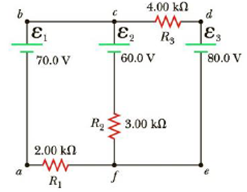Chapter 18, Problem 25P

Chapter
Section
Textbook Problem

Using Kirchhoff’s rules (a) find the current in each resistor shown in Figure P18.25 and (b) find the potential difference between point c and f.Figure P18.25

(a)

To determine
The current in each resistor I1,I2,I3

Explanation

Given Info:

Resistance R1 is 2.00 , Resistance R2 is 3.00 , Resistance R3 is 4.00 , Voltage ε1 is 70.0V , Voltage ε2 is 60.0V , and Voltage ε3 is 80.0V .

Explanation:

The below figure shows the direction of current flow in the circuit.

• ε1,ε2,ε3 is voltage in the circuits
• R1,R2,R3 is corresponding resistance in the circuits
• I1,I2,I3 is corresponding current in the circuits

Apply Kirchhoff’s voltage law to the left and right loop.

From the left loop abcfa,

ε1ε2R1I1R2I2=0

Substitute, 2.00 for R1 , 3.00 for R2 , 70.0V for ε1 and 60.0V for ε2 , in the above equation to find expression for abcfa

70.0V60.0V(2.00)I1(3.00)I2=010.0V(3.00)I2=2.00I15.0V(1.50)I2=I1 (I)

From the right loop edcfe,

ε3ε2R2I2R3I3=0

Substitute, 3.0 for R2 , 4.0 for R3 60.0V for ε2 and 80.0V for ε3 in the above equation to find expression for edcfe,

80.0V60.0V4.00I33.00I2=020.0V4.00I33.00I2=020.0V3.00I2=4.00I35.0V0.75I2=I3 (II)

Conclusion:

Formula to calculate current by using Kirchhoff’s junction rule

I2=I1+I3

Use, 5

(b)

To determine
The potential difference between point c and f.

Still sussing out bartleby?

Check out a sample textbook solution.

See a sample solution

The Solution to Your Study Problems

Bartleby provides explanations to thousands of textbook problems written by our experts, many with advanced degrees!

Get Started

Find more solutions based on key concepts